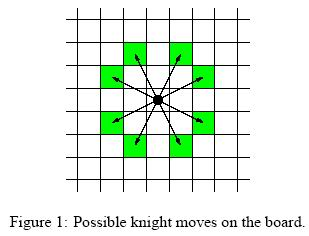poj1915,

## Problem:

Knight Moves
 Time Limit: 1000MS Memory Limit: 30000K Total Submissions: 14965 Accepted: 6680
Description
Background
Mr Somurolov, fabulous chess-gamer indeed, asserts that no one else but him can move knights from one position to another so fast. Can you beat him?
The Problem
Your task is to write a program to calculate the minimum number of moves needed for a knight to reach one point from another, so that you have the chance to be faster than Somurolov.
For people not familiar with chess, the possible knight moves are shown in Figure 1.Input
The input begins with the number n of scenarios on a single line by itself.
Next follow n scenarios. Each scenario consists of three lines containing integer numbers. The first line specifies the length l of a side of the chess board (4 <= l <= 300). The entire board has size l * l. The second and third line contain pair of integers {0, ..., l-1}*{0, ..., l-1} specifying the starting and ending position of the knight on the board. The integers are separated by a single blank. You can assume that the positions are valid positions on the chess board of that scenario.
Output
For each scenario of the input you have to calculate the minimal amount of knight moves which are necessary to move from the starting point to the ending point. If starting point and ending point are equal,distance is zero. The distance must be written on a single line.
Sample Input
```3
8
0 0
7 0
100
0 0
30 50
10
1 1
1 1```
Sample Output
```5
28
0```
Source
TUD Programming Contest 2001, Darmstadt, Germany

## Solution:

PS: don't use vector operation here, or you will get TLE.

## Source Code:

//Fri Apr  8 18:53:31 CDT 2011
#include <vector>
#include <list>
#include <map>
#include <set>
#include <deque>
#include <queue>
#include <stack>
#include <bitset>
#include <algorithm>
#include <functional>
#include <numeric>
#include <utility>
#include <sstream>
#include <iostream>
#include <iomanip>
#include <cstdio>
#include <cmath>
#include <cstdlib>
#include <cctype>
#include <string>
#include <cstring>
#include <cstdio>
#include <cmath>
#include <cstdlib>
#include <ctime>

using namespace std;

class Square {
public:
int x;
int y;
int cost;
bool visited;
};

int xx[] = { -2, +2, -1, +1, +1, -1, +2, -2 };
int yy[] = { -1, +1, -2, +2, -2, +2, -1, +1 };
int dir = { { -1, -2 }, { 1, -2 }, { -2, -1 }, { 2, -1 }, { -2, 1 }, { 2,
1 }, { -1, 2 }, { 1, 2 } };

Square chess;
queue<Square> q;

void init(int N) {
while (q.empty() == false)
q.pop();
for (int i = 0; i < N; i++) {
for (int j = 0; j < N; j++) {
chess[i][j].x = i;
chess[i][j].y = j;
chess[i][j].cost = 0;
chess[i][j].visited = false;
}
}
//  for (int i = 0; i < 8; i++)
//      for (int j = 0; j < 8; j++)
//          cout << "Chess at " << chess[i][j].x << chess[i][j].y << ": "
//                  << chess[i][j].cost << ", visited: "
//                  << (chess[i][j].visited == true ? 1 : 0) << endl;
}

bool checkBound(int x, int y, int N) {
if (x >= 0 && x < N && y >= 0 && y < N)
return true;
return false;
}

int bfs(int a, int b, int c, int d, int N) {
int x = a;
int y = b;
q.push(chess[x][y]);
while (q.empty() == false) {
Square tmp = q.front();
q.pop();
x = tmp.x;
y = tmp.y;
if (x == c && y == d)
return chess[x][y].cost;
if (chess[x][y].visited == false) {
chess[x][y].visited = true;
for (int i = 0; i < 8; i++) {
int x1 = x + dir[i];
int y1 = y + dir[i];
if (checkBound(x1, y1, N)) {
chess[x1][y1].cost = 1 + chess[x][y].cost;
q.push(chess[x1][y1]);
}
}
}
}
return 0;
}

int main(int argc, const char* argv[]) {
//freopen("input.in", "r", stdin);
int T;
scanf("%d", &T);
int a, b;
int c, d;
int N;
while (T--) {
scanf("%d%d%d%d%d", &N, &a, &b, &c, &d);
//cin >> N;
//cin >> a >> b;
//cin >> c >> d;
init(N);
printf("%d\n", bfs(a, b, c, d, N));
}
//fclose(stdin);
return 0;
}